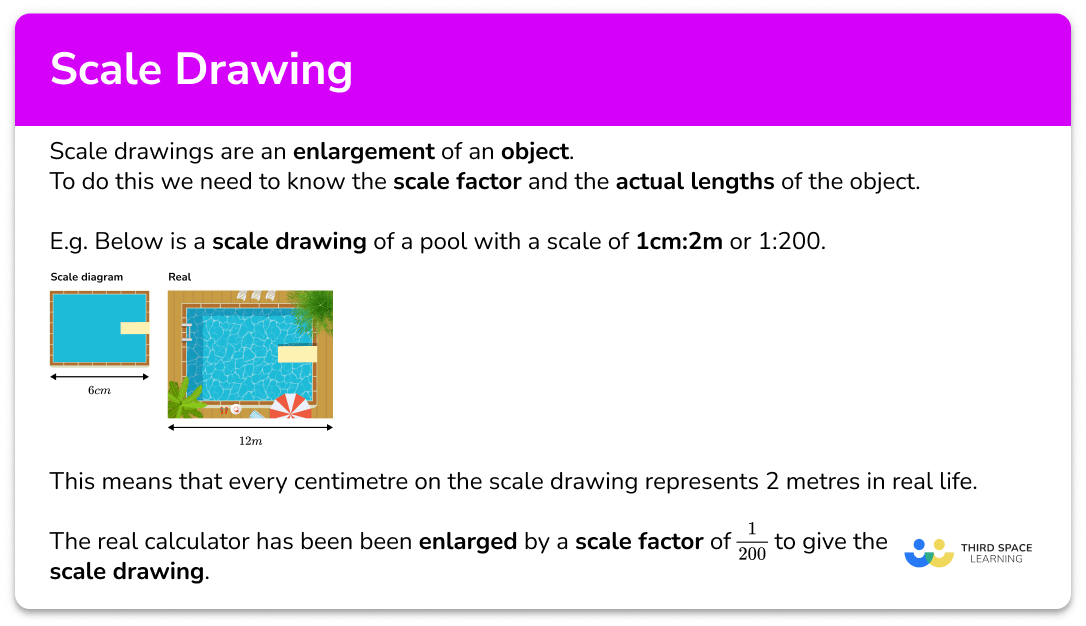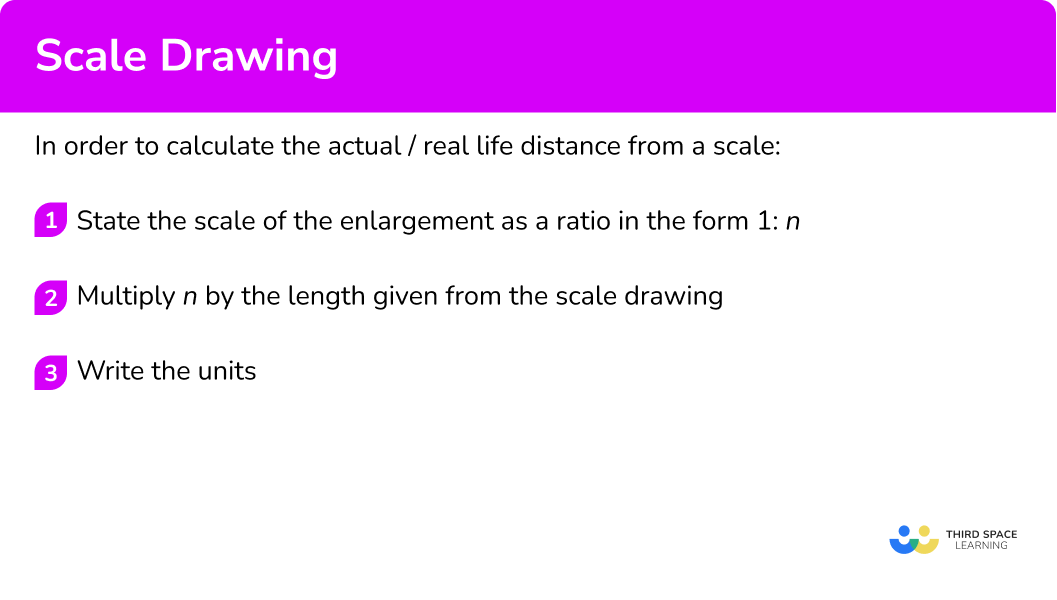# Scale Drawing

Here we will learn about scale drawings, including creating scale drawings, using scale factors, and word problems.

There are also scale diagrams and drawings worksheets based on Edexcel, AQA and OCR exam questions, along with further guidance on where to go next if you’re still stuck.

## What is a scale drawing?

A scale drawing is an enlargement of an object.

An enlargement changes the size of an object by multiplying each of the lengths by a scale factor to make it larger or smaller.

The scale of a drawing is usually stated as a ratio.

E.g.

1cm:5m

This is said as “ 1 centimetres to 5 metres” and means every 1 centimetre on the diagram represents 5 metres in real life.

In order to interpret and produce scale drawings we need to know the scale factor and the actual lengths of the object.

E.g.

Below is a scale drawing of a pool with a scale of \bf{1cm:2m} or 1:200.

This means that every centimetre on the diagram represents 2 metres in real life.

So the 6cm width of the diagram represents a 12m width on the real pool.

The real pool has been been enlarged by a scale factor of \frac{1}{200} to give the scale drawing.

The majority of scale drawing questions will involve polygons or 2D drawings such as floor plans or using a map although there is overlap with topics such as enlargement, construction of triangles, and loci

### What is a scale drawing?## How to calculate the actual / real life distance from a scale

In order to calculate the actual/real life distance from a scale:

1. State the scale of the enlargement as a ratio in the form \bf{1:n} .
2. Multiply \bf{n} by the length given from the scale drawing.
3. Write the units.

### Explain how to calculate the actual / real life distance from a scale## Related lessons on scale

Scale drawing is part of our series of lessons to support revision on scale. You may find it helpful to start with the main scale lesson for a summary of what to expect, or use the step by step guides below for further detail on individual topics. Other lessons in this series include:

## Scale drawing examples

### Example 1: map

A map has a scale of 1cm:2km. Find the actual distance represented by 5cm on the map.

1. State the scale of the enlargement as a ratio in the form \bf{1:n} .

1cm:2km

2Multiply \bf{n} by the length given from the scale drawing.

2 \times 5=10

3Write the units.

10km.

### Example 2: plan of a building

A plan of a block of flats has the scale 2.5cm:800m. What is the real distance represented by 5.7cm on the plan?

Dividing both sides by 2.5, we get 1cm:320m

320 \times 5.7=1 \ 824

1 \ 824m

### Example 3: atlas

A world atlas has a scale of 2cm:5 \ 000km. Calculate the real world distance that is represented by 23mm on the atlas.

As the length required is in millimetres, we first need to change the 2cm to millimetres.

2cm:5 \ 000km = 20mm:5 \ 000km

The we can reduce the ratio so we have 1 \ mm:n \ km. Dividing both sides by 20, we get:

1mm:250km

250 \times 23=5750

5750km

## How to calculate the scale drawing distance from a scale

In order to calculate the scale drawing distance from a scale:

1. State the scale of the enlargement as a ratio in the form \bf{1:n} .
2. Divide the real life distance by the scale ratio.
3. Write the units.

## Calculate scale drawing distance examples

### Example 4: model object

A model car is made using the model to an actual distance of 1cm:40cm. The height of the car is 170cm. Calculate the height of the model car.

The ratio given is 1:40

170 \div 40=4.25

4.25cm

### Example 5: floor plan

A plan of a kitchen uses the scale 1cm:0.2m. Calculate the distance on the plan for the actual distance of 5.62m.

The ratio is already in the form 1:n .

1cm:0.2m

5.62 \div 0.2=28.1

28.1cm

### Example 6: map of the UK

A map of the UK is drawn using the scale 50cm:1400km. Calculate how far 86.8km would be on the map.

Let’s convert the ratio to the form 1 \ cm:n \ km by dividing both sides of the ratio by 50.

50cm:1400km=1cm:28km

Now we can calculate the length on the map

86.8 \div 28=3.1

3.1cm

### Common misconceptions

• Multiplying the real life distance by the ratio scale

The real life distance is multiplied by the ratio scale, giving an incorrect distance on the plan.

• Dividing the model / plan / map distance by the ratio scale

The distance on the model / plan / map is divided by the ratio scale meaning that the real life distance is incorrect.

• Ratio scale not simplified

If given the ratio 2cm:5km, it is easier to calculate when the ratio is in the form 1:n and so we must find an equivalent ratio before using the scale.

Here the ratio would be 1cm:2.5km so 1cm on the map would be equal to 2.5km in real life.

• Incorrect units in the solution

The units for the model are mixed up with the units for the real life distance. For example if we were calculating the distance of 10cm on a map with the scale ratio of 1cm:5km, \ 10 \times 5=50cm is stated whereas the correct solution would be 10 \times 5=50km.

• Converting units

Sometimes the units need to be converted so that you are working with the same units, making calculations much easier to comprehend. Make sure you know how to convert between different metric units. For example, the map scale is given as 1:25 \ 000 which means that 1cm on the map is equivalent to 25 \ 000cm in real life.

If the answer is asked to be written in kilometres, the real life value in centimetres must be divided by 100 \ 000 to get the same measurement in kilometres. 1:25 \ 000 = 1cm:0.25km

### Practice scale drawing questions

1. A map has a scale of 1cm:3km. How far is the actual distance of 3.5cm on the map?

0.86km0.86cm10.5km10.5cm3 \times 3.5=10.5km

2. An atlas has the scale 2cm:500km. Calculate the real distance for a length of 15cm on the map.

3750km7500km33.3km3750cm\begin{aligned} &2cm:500km = 1cm:250km \\\\ &250 \times 15=3750km \end{aligned}

3. A plan of a bridge uses the scale 10cm:18.2m. The height of the bridge on the plan is 5.9cm. Calculate the real life height of the bridge.

10.738m10.738cm107.38m3.08m\begin{aligned} &1cm:1.82m \\\\ &1.82 \times 5.9=10.738m \end{aligned}

4. A model elephant is created using a scale of 10cm:2.8m. A distance of 138.8cm is taken from the real life elephant. How long is this length on the model?

0.38864cm0.38864m4.96cm4.96m\begin{aligned} &138.8cm=1.388m \\\\ &10cm:2.8m=1cm:0.28m \\\\ &1.388 \div 0.28=4.96cm \end{aligned}

5. A map of the UK uses the scale 8m:1400km. Calculate the distance on the map that represents the actual length of 132km.

1.326m13.26m0.754km0.754m\begin{aligned} &8m:1400km=1m:175km \\\\ &132 \div 175=0.754m \ (3dp) \end{aligned}

6. The plan of a garage is drawn using the drawing to actual scale of 0.9cm:20cm. What is the length of the garage on the plan, if it is 5.6m in real life?

28cm6.22m6.22cm25.2cm0.9cm:20cm=1cm:22.\dot{2}cm

Model to actual ratio is therefore 1cm:22.\dot{2}cm

\begin{aligned} &5.6m=560cm \\\\ &560 \div 22. \dot{2}=25.2cm \end{aligned}

### Scale drawing GCSE questions

1. A map has a scale of 1cm:0.5km. A dog walker walks around 18km per day. Calculate how far they will have walked on a map.

(2 marks)

18 \div 0.5

(1)

=36cm

(1)

2. (a) The distance between two cities in an American State is 146.8km. The distance between the two cities on a map is 29.36cm. Calculate the scale ratio of the map to the actual distance in the form 1cm:nkm.

(b) The river that travels through the state is measured on the plan to be 45cm. Calculate the actual length of the river. State the units in your solution.

(4 marks)

(a)

146.8 \div 29.36=

(1)

1cm:5km

(1)

(b)

45 \times 5=225

(1)

km

(1)

3. Below is the map of a desert island.Using the scale provided, calculate the distance between the Palm Trees and the Waterfall.

(3 marks)

Distance [6.3-6.7cm]

(1)

Their 6.5 \times 50

(1)

325m

(1)

## Learning checklist

You have now learned how to:

• Multiplying and dividing by powers of 10 in scale drawings or by multiplying and dividing by powers of a 1,000 in converting between units such as kilometres and metres
• Solve problems involving similar shapes where the scale factor is known or can be found

## Still stuck?

Prepare your KS4 students for maths GCSEs success with Third Space Learning. Weekly online one to one GCSE maths revision lessons delivered by expert maths tutors.

Find out more about our GCSE maths tuition programme.